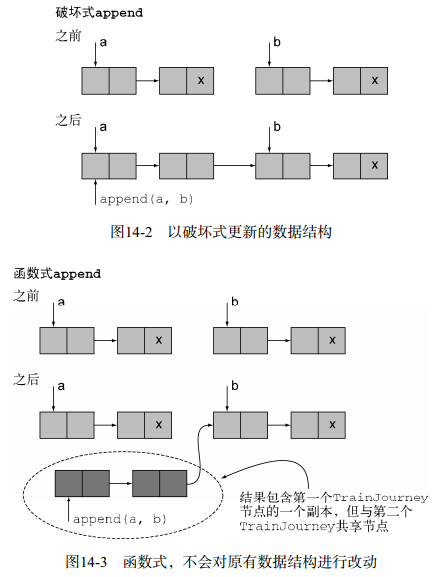# Java8 In Action-3.超越 Java 8 (二)

2.函数式编程的技巧
2.1无处不在的函数
“函数式编程”意指函数或者方法的行为应该像“数学函数”一样——没有任何副作用。让函数可以像任何其他值一样随意使用：可以作为参数传递，可以作为返回值，还能存储在数据结构中。能够像普通变量一样使用的函数称为一等函数（first-class function）。这是Java 8补充的全新内容：通过::操作符，你可以创建一个方法引用，像使用函数值一样使用方法，也能使用Lambda表达式（比如， (int x) -> x + 1）直接表示方法的值。 Java 8中使用下面这样的方法引用将一个方法引用保存到一个变量是合理合法的：

	    Function<String,Integer> strToIntFun = Integer::parseInt;
Integer apply = strToIntFun.apply("123");
System.out.println(apply);


 接受至少一个函数作为参数
 返回的结果是一个函数

        Function<User, Integer> getAgeFun = User::getAge;
//Comparator.comparing就是一个高阶函数
Comparator<User> comparator = Comparator.comparing(getAgeFun);
UserContractorFun<String,String,Integer,User> userFun = User::new;
List<User> userList = Arrays.asList(userFun.apply("a","f",27),userFun.apply("b","m",18),userFun.apply("c","m",21));
userList.sort(comparator);
userList.forEach(System.out::println);

@FunctionalInterface
public interface UserContractorFun<T,R,U,User> {
User apply(T t,R r,U u);
}

public class User {
private String name;
private String sex;
private Integer age;

public User(String name, String sex, Integer age) {
this.name = name;
this.sex = sex;
this.age = age;
}

getter/setter()...

@Override
public String toString() {
return "User{" +
"name='" + name + '\'' +
", sex='" + sex + '\'' +
", age=" + age +
'}';
}
}


        /**
* 科里化
* 应用场景:不同货币的的单位转换,摄氏度与华氏度的转换
* 遵循下面的模式:
* (1) 乘以转换因子
* (2) 如果需要，进行基线调整
*/
DoubleUnaryOperator convertCtoF = curriedConverter(9.0/5, 32);
DoubleUnaryOperator convertUSDtoGBP = curriedConverter(0.6, 0);
DoubleUnaryOperator convertKmtoMi = curriedConverter(0.6214, 0);
double gbp = convertUSDtoGBP.applyAsDouble(1000);

/**
* @param x 待转换值
* @param f 转换因子
* @param b 基准值
* @return
*/
public static double converter(double x,double f,double b){
return x*f + b;
}

/**
* 不一次性传递所有的参数x、f和b,相反，只使用参数f和b并返回了另一个方法，
* 这个方法会接收参数x，最终返回你期望的值x * f + b.
* 通过这种方式，你复用了现有的转换逻辑，同时又为不同的转换因子创建了不同的转换方法。
* @param f
* @param b
* @return
*/
public static DoubleUnaryOperator curriedConverter(double f, double b){
return (double x) -> x * f + b;
}


2.2持久化数据结构2.3 Stream 的延迟计算
Stream是处理数据集合的利器。不过，由于各种各样的原因，包括实现时的效率考量， Java 8的设计者们在将Stream引入时采取了比较特殊的方式。其中一个比较显著的局限是，你无法声明一个递归的Stream，因为Stream仅能使用一次。

public interface MyList<T> {
T head();
MyList<T> tail();
default boolean isEmpty() {
return true;
}
MyList<T> filter(Predicate<T> p);
}

package com.h.java8;

import java.util.function.Predicate;
import java.util.function.Supplier;

/**
* Created by John on 2018/10/4.
*/
class LazyList<T> implements MyList<T> {
final T head;
final Supplier<MyList<T>> tail;

public LazyList(T head, Supplier<MyList<T>> tail) {
this.head = head;
this.tail = tail;
}

@Override
public T head() {
return head;
}

@Override
public MyList<T> tail() {
/**
* 这里tail使用了一个Supplier方法提供了延迟性
* 调用Supplier的get方法会触发延迟列表（LazyList）的节点创建，就像工厂会创建新的对象一样。
*/
return tail.get();
}

@Override
public boolean isEmpty() {
return false;
}

/**
* 延迟筛选器
* @param p
* @return
*/
@Override
public MyList<T> filter(Predicate<T> p) {
return isEmpty() ?
this :   //你可以返回一个新的Empty<>()，不过这和返回一个空对象的效果是一样的
p.test(head()) ?
new LazyList<>(head(), () -> tail().filter(p)) :
tail().filter(p);
}
}

/**
* 延迟计算(非限制式计算):
* 生成质数,只在你需要处理质数的那个时刻（比如，要调用方法limit了）才对Stream进行计算。
*/
LazyList<Integer> lazyList = from(2);
Integer two = lazyList.head();
Integer three = lazyList.tail().head();
Integer four = lazyList.tail().tail().head();
//实时计算得出 2,3,4
System.out.println(two + " " + three + " " + four);
System.out.println("=================");
LazyList<Integer> numbers = from(2);
int two2 = primes(numbers).head();
int three2 = primes(numbers).tail().head();
int five2 = primes(numbers).tail().tail().head();
System.out.println(two2 + " " + three2 + " " + five2);
System.out.println("+++++++++++++++++++");
printAll(primes(numbers));

public static <T> void printAll(MyList<T> list){
while (!list.isEmpty()){
System.out.println(list.head());
list = list.tail();
}
}

static <T> void printAll2(MyList<T> list){
if (list.isEmpty())
return;
System.out.println(list.head());
printAll(list.tail());
}

/**
* 质数筛选器
* @param numbers
* @return
*/
public static MyList<Integer> primes(MyList<Integer> numbers) {
return new LazyList<>(
numbers.head(),
() -> primes(
numbers.tail()
.filter(n -> n % numbers.head() != 0)
)
);
}

/**
* 延迟列表
* @param n
* @return
*/
public static LazyList<Integer> from(int n) {
return new LazyList(n, () -> from(n+1));
}


2.4 模式匹配

Java语言中还有另一种方式可以解包数据类型，那就是使用访问者（Visitor）设计模式。本质上，使用这种方法你需要创建一个单独的类，这个类封装了一个算法，可以“访问”某种数据类型。

class BinOp extends Expr{
...
public Expr accept(SimplifyExprVisitor v){
return v.visit(this);
}
}
//SimplifyExprVisitor现在就可以访问BinOp对象并解包其中的内容了：
public class SimplifyExprVisitor {
...
public Expr visit(BinOp e){
if("+".equals(e.opname) && e.right instanceof Number && …){
return e.left;
}
return e;
}
}


Java中的伪模式匹配

public class Expr {

}

class Number extends Expr {
public Number(int val) {
this.val = val;
}

int val;
}

class BinOp extends Expr {
public BinOp(String opname, Expr left, Expr right) {
this.opname = opname;
this.left = left;
this.right = right;
}

String opname;
Expr left, right;
}

@FunctionalInterface
public interface TriFunction<S, T, U, R>{
R apply(S s, T t, U u);
}

	    Expr e = new BinOp("+", new Number(5), new Number(0));
Expr match = simplify(e);
System.out.println(((Number)match).val);//5

public static Expr simplify(Expr e) {
TriFunction<String, Expr, Expr, Expr> binopcase = //处理BinOp表达式
(opname, left, right) -> {
if ("+".equals(opname)) {
if (left instanceof Number && ((Number) left).val == 0) {
return right;
}
if (right instanceof Number && ((Number) right).val == 0) {
return left;
}
}
if ("*".equals(opname)) {
if (left instanceof Number && ((Number) left).val == 1) {
return right;
}
if (right instanceof Number && ((Number) right).val == 1) {
return left;
}
}
return new BinOp(opname, left, right);
};
Function<Integer, Expr> numcase = val -> new Number(val);
// 如果用户提供的Expr无法识别时进行的默认处理机制
Supplier<Expr> defaultcase = () -> new Number(0);
return patternMatchExpr(e, binopcase, numcase, defaultcase);//进行模式匹配
}

public static <T> T patternMatchExpr(
Expr e,
TriFunction<String, Expr, Expr, T> binopcase,
Function<Integer, T> numcase,
Supplier<T> defaultcase) {
return
(e instanceof BinOp) ?
binopcase.apply(((BinOp)e).opname, ((BinOp)e).left,
((BinOp)e).right) :
(e instanceof Number) ?
numcase.apply(((Number)e).val) :
defaultcase.get();
}

public static <T> void printAll(MyList<T> list){
while (!list.isEmpty()){
System.out.println(list.head());
list = list.tail();
}
}


2.5 杂项

System.out.println(repeat(3, (Integer x) -> 2*x).apply(10));//80

/**
* repeat(3, (Integer x) -> 2*x);效果:x ->(2*(2*(2*x)))
* @param n
* @param f
* @param <A>
* @return
*/
public static <A> Function<A,A> repeat(int n, Function<A,A> f) {
return n==0 ? x -> x
: compose(f, repeat(n-1, f));
}

/**
* 函数功能:接受函数f和g作为参数，并返回一个函数，实现的效果是先做f，接着做g。
* 可以接着用这种方式定义一个操作，通过结合器完成内部迭代的效果。
* @param g
* @param f
* @param <A>
* @param <B>
* @param <C>
* @return
*/
public static <A,B,C> Function<A,C> compose(Function<B,C> g, Function<A,B> f) {
return x -> g.apply(f.apply(x));
}


2.6 小结
 一等函数是可以作为参数传递，可以作为结果返回，同时还能存储在数据结构中的函数。
 高阶函数接受至少一个或者多个函数作为输入参数，或者返回另一个函数的函数。 Java中典型的高阶函数包括comparing、 andThen和compose。
 科里化是一种帮助你模块化函数和重用代码的技术。
 持久化数据结构在其被修改之前会对自身前一个版本的内容进行备份。因此，使用该技术能避免不必要的防御式复制。
 Java语言中的Stream不是自定义的。
 延迟列表是Java语言中让Stream更具表现力的一个特性。延迟列表让你可以通过辅助方法（supplier）即时地创建列表中的元素，辅助方法能帮忙创建更多的数据结构。
 模式匹配是一种函数式的特性，它能帮助你解包数据类型。它可以看成Java语言中switch语句的一种泛化。
 遵守“引用透明性”原则的函数，其计算结构可以进行缓存。
 结合器是一种函数式的思想，它指的是将两个或多个函数或者数据结构进行合并。09-10537
09-241139
02-12954
11-06232
04-03228
03-30675
02-1520
11-06169
07-01694
07-1719
05-0788
03-28411
©️2020 CSDN 皮肤主题: 技术黑板 设计师:CSDN官方博客